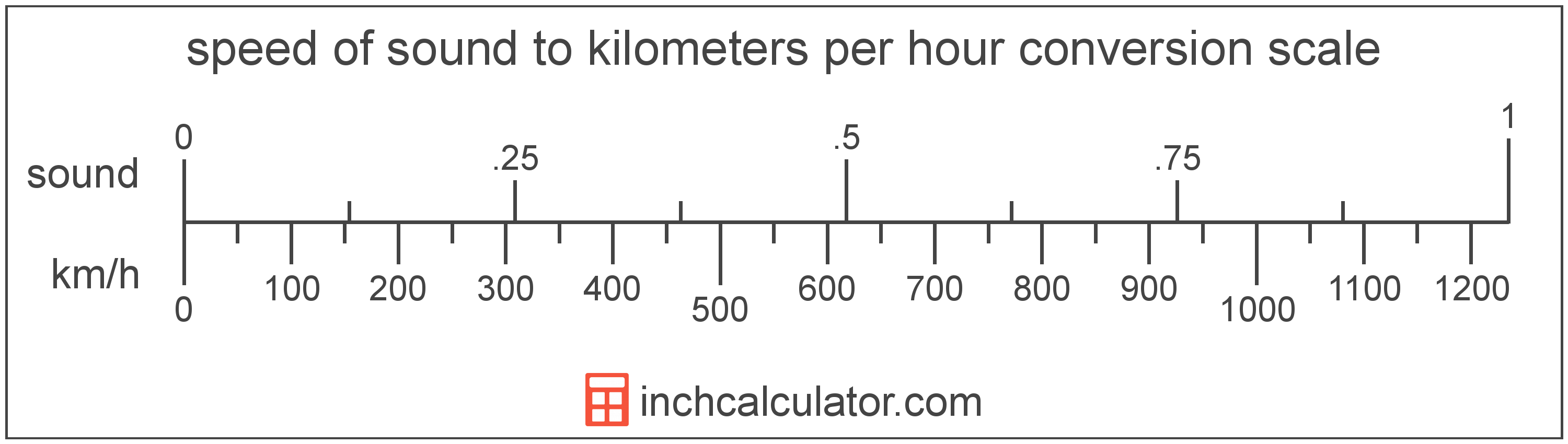# Speed of Sound to Kilometers per Hour Converter

Enter the speed in speed of sound below to get the value converted to kilometers per hour.

Results in Kilometers per Hour:1 sound = 1,234.8 km/h

Do you want to convert kilometers per hour to speed of sound?

## How to Convert Speed of Sound to Kilometers per Hour

To convert a measurement in speed of sound to a measurement in kilometers per hour, multiply the speed by the following conversion ratio: 1,234.8 kilometers per hour/speed of sound.

Since one speed of sound is equal to 1,234.8 kilometers per hour, you can use this simple formula to convert:

kilometers per hour = speed of sound × 1,234.8

The speed in kilometers per hour is equal to the speed in speed of sound multiplied by 1,234.8.

For example, here's how to convert 5 speed of sound to kilometers per hour using the formula above.
kilometers per hour = (5 sound × 1,234.8) = 6,174 km/hSpeed of sound and kilometers per hour are both units used to measure speed. Keep reading to learn more about each unit of measure.

## What Is the Speed of Sound?

The speed of sound is the distance a sound wave travels through an elastic medium. The speed of sound through air at 20 °C is equal to 343 meters per second, or roughly 767 miles per hour.

Speed of sound can be abbreviated as sound; for example, 1 speed of sound can be written as 1 sound.

## What Are Kilometers per Hour?

Kilometers per hour are a measurement of speed expressing the distance traveled in kilometers in one hour.

The kilometer per hour, or kilometre per hour, is an SI unit of speed in the metric system. Kilometers per hour can be abbreviated as km/h, and are also sometimes abbreviated as kph. For example, 1 kilometer per hour can be written as 1 km/h or 1 kph.

In the expressions of units, the slash, or solidus (/), is used to express a change in one or more units relative to a change in one or more other units. For example, km/h is expressing a change in length or distance relative to a change in time.

Kilometers per hour can be expressed using the formula:
vkm/h = dkm / thr

The velocity in kilometers per hour is equal to the distance in kilometers divided by time in hours.

## Speed of Sound to Kilometer per Hour Conversion Table

Table showing various speed of sound measurements converted to kilometers per hour.
Speed Of Sound Kilometers Per Hour
0.001 sound 1.2348 km/h
0.002 sound 2.4696 km/h
0.003 sound 3.7044 km/h
0.004 sound 4.9392 km/h
0.005 sound 6.174 km/h
0.006 sound 7.4088 km/h
0.007 sound 8.6436 km/h
0.008 sound 9.8784 km/h
0.009 sound 11.11 km/h
0.01 sound 12.35 km/h
0.02 sound 24.7 km/h
0.03 sound 37.04 km/h
0.04 sound 49.39 km/h
0.05 sound 61.74 km/h
0.06 sound 74.09 km/h
0.07 sound 86.44 km/h
0.08 sound 98.78 km/h
0.09 sound 111.13 km/h
0.1 sound 123.48 km/h
0.2 sound 246.96 km/h
0.3 sound 370.44 km/h
0.4 sound 493.92 km/h
0.5 sound 617.4 km/h
0.6 sound 740.88 km/h
0.7 sound 864.36 km/h
0.8 sound 987.84 km/h
0.9 sound 1,111 km/h
1 sound 1,235 km/h

## References

1. CK-12, Speed of Sound, https://www.ck12.org/physics/speed-of-sound/lesson/Speed-of-Sound-MS-PS/
2. National Institute of Standards and Technology, NIST Guide to the SI, Chapter 6: Rules and Style Conventions for Printing and Using Units, https://www.nist.gov/pml/special-publication-811/nist-guide-si-chapter-6-rules-and-style-conventions-printing-and-using